Grade 10 questions on how to simplify radicals expressions with solutions are presented.

In order to simplify radical expressions, you need to be aware of the following rules and properties of radicals

1) From definition of
n th root(s) and principal rootExamplesMore examples on
Roots of Real Numbers and Radicals .
2) Product (Multiplication) formula of radicals with equal indices is given byMore examples on how to

3) Quotient (Division) formula of radicals with equal indices is given byMore examples on how to

ExampleMore examples on how to

5) You may rewrite expressions without radicals (to rationalize denominators) as follows
A) Example 1:B) Example 2:C) Example 3:More examples on how to
Rationalize Denominators of Radical Expressions .

Examples
Rationalize and simplify the given expressions1)
Write 128 and 32 as product/powers of prime factors: 128 = 2
7 , 32 = 2 5 hence2)
Use product rule to write that √2 √6 = √123)
Write 14 and 63 as products of prime numbers 14 = 2 � 7 , 63 = 3
2 � 7 and substitute4)
Write 32 and 16 as products of prime numbers 32 = 2
5 , 16 = 2 4 and substitute5)
Write 64 as products of prime numbers 64 = 2
6 and substituteRationalize the denominator by multiplying numerator and denominator by (
3 √7) 26)
Write 54 as products of prime numbers 54 = 2 � 3
3 and substitute7)
Multiply the denominator and numerator by the conjugate of the denominatorExpand and simplifyUse all the rules and properties of radicals to rationalize and simplify the following expressions.Solutions to the Above Questions

1. Write 25 and 125 as the product of prime factors: 25 = 52 and 125 = 53, hence2. Write 64 and 16 as the product of prime factors: 64 = 26 and 16 = 24, hence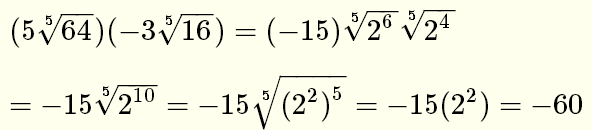3. Use product rule4. Convert the mixed number under the radical into a fraction and substituteUse the division formula for radicalsWrite 64 and 27 as product of prime factors, substitute and simplify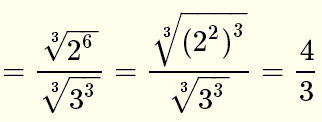5. Use the product formula and write 34 as the product of prime factorsSimplify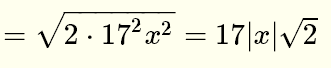For √(17 x) and √(34 x) to be real numbers, x must be positive hence |x| = x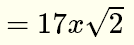6. Write the radicand as a square and simplify7. Write the radicand as the product of $2$ and a square and simplify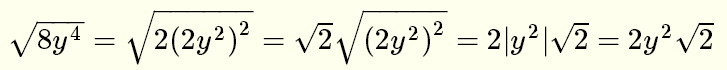Write as the product of prime factors and simplify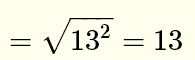9. Since n is a positive integer, then N = 2 n + 1 is an odd integer. Hence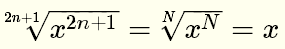10. Since n is a positive integer, then N = 2 n is an even integer. Hence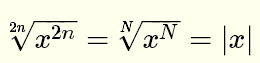11.12. Use division rule and simplify the radicand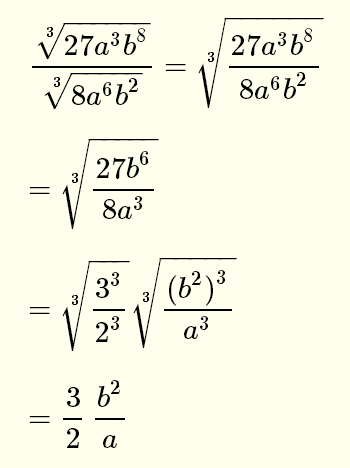13. Multiply numerator and denominator by the conjugate of the denominatorExpand and simplifyMore
High School Math (Grades 10, 11 and 12) - Free Questions and Problems With Answers
More
Middle School Math (Grades 6, 7, 8, 9) - Free Questions and Problems With Answers
More
Primary Math (Grades 4 and 5) with Free Questions and Problems With Answers
Author -